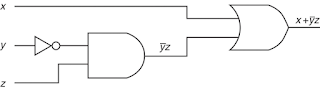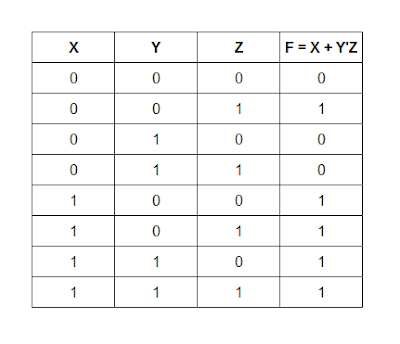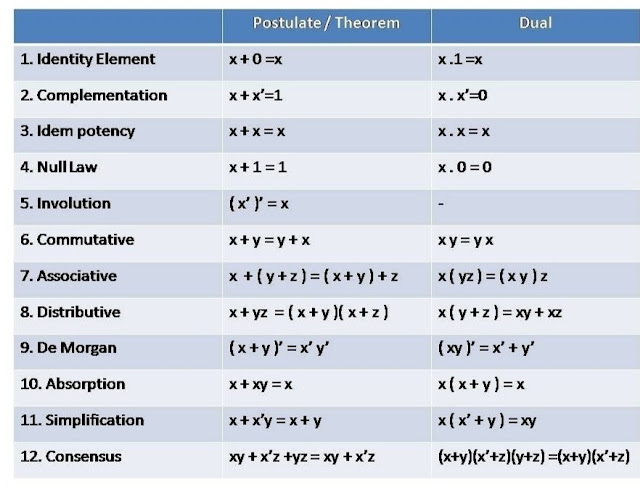# Laws of Boolean Algebra

Boolean Algebra is an algebra that deals with binary variables and logic operations. The three basic logic operations are AND, OR and complement.

## Boolean Algebra

Boolean Algebra is an algebra that deals with binary variables and logic operations. The three basic logic operations are AND, OR and complement. A Boolean function can be expressed algebraically with binary variables, the logic operations symbols parentheses and equal sign. For a given value of the variables, the Boolean function can be either 1 or 0.

Consider, for example, the Boolean function

F = x + y' z

The logic diagram for F is shown in Fig.Logic diagram

The function F is equal to 1 if x is 1 or if both y' and z are equal to 1; F is equal to 0 otherwise. But saying that y'=1 is equivalent to saying that y'=0 then  since y' is the complement of y. The relationship between the functions and its binary variables can be represented in a truth table. To represent a function in a truth table we need a list of the 2combinations of the n binary variables. There are 8 possible distinct combinations for assigning bits to the three variables x, y and z.Truth table

## Laws of Boolean Algebra

A set of rules or Laws of Boolean Algebra expressions have been invented to help reduce the number of logic gates needed to perform a particular logic operation resulting in a list of functions or theorems known commonly as the Laws of Boolean Algebra.

A Boolean function can be transformed from an algebraic expression into a logic diagram composed of AND, OR and inverter Gates. The purpose of Boolean Algebra is to facilitate the analysis and design of Digital circuits. It provides a convenient tool to-

1. Express  in algebraic form a truth table relationship between binary variables.
2. Express  in algebraic form the input-output relationship of logic diagrams.
3. Find simpler circuits for the same function.

Boolean function is specified by a truth table can be expressed algebraically in many different ways. By manipulating a boolean expressions according to boolean algebra rules, one may obtain a simpler expressions that will require fewer gates. To see how this is done, we must first study the manipulative capabilities of boolean algebra. Boolean algebra calculator can be used to solve the problems of boolean algebra according to its laws.Basic Identities of Boolean Algebra

All the identities in the table can be proven by means of truth tables. These identities apply to single variables or to Boolean functions expressed in terms of binary variables.